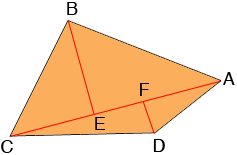SEARCH HOMEMath Central Quandaries & QueriesQuestion from Joel, a student: Diagonal ac of quadrilateral ABCD is 60cm and the lengths of perpendiculars to It from the opposite vertices are 4.2cm and 5.8 cm find the area of the quadrilateral ABCDHi Joel,

Is this for a convex quadrilateral? If so then here is my diagram.$BE$ and $DF$ are perpendicular to $AC$ and $BE$ has length 5.8 cm and $DF$ has length 4.2 cm.

The area of the quadrilateral $ABCD$ is the sum of the areas of the triangles $ABC$ and $ACD.$ What are the areas of the triangles $ABC$ and $ACD?$

PennyMath Central is supported by the University of Regina and the Imperial Oil Foundation.